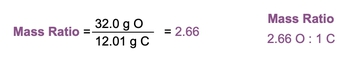## General Chemistry

Learn the toughest concepts covered in Chemistry with step-by-step video tutorials and practice problems by world-class tutors

2. Atoms & Elements

# Law of Definite Proportions

"Different samples of a pure chemical compound always contain the same proportions of elements by mass."

Proust's Law of Definite Proportions
1
concept

## Law of Definite Proportions2m
Play a video:
Let's examine the law definite proportions. In 17 99 the French chemist Joseph L. Promised originated the law of definite proportions because of its immense contributions to it. It's sometimes referred to simply ask Proust law, but it also goes by the law of constant or definite composition. The law itself uses what we call mass ratios. These mass ratios are fractions or proportions of elements by Mass. The whole concept behind a lot of definite proportions is that different samples of a cure chemical compound always contained the same proportion development by mass. So let's say, for example, that I take ah sample from New York City, and I suspect that it's CO two and I take a sample of in another city, London, England. And I suspect that CO two, if we're following the law of different proportions, both samples should have the same mass ratio. Now, for the mass ratio, we're gonna place the element with the larger mass on top, not going back to our whole idea of co two in two different cities. Remember, Seal to itself is composed of one carbon and two oxygen, and we know that if we look at the periodic table. The atomic massive carbon is 12.1 g per mole in the massive, oxygen is around 16 g per mole. When you multiply the number of each element by atomic Mass, we'll see how much of it contributes to the old raw mass of CO. Two. So carbon itself contributes 12.1 g total, and oxygen contributes 32 g total. Not using the mass ratio, we place the larger mass on top, which is the 32 g of oxygen in the smaller mass on the bottom. When we divide those two numbers, that gives me approximately 2 66. What that 2.66 is telling me is that we have 2. oxygen for everyone. Carbon. This would be our mass ratio. And if our examining two samples of CO. 21 from New York City and one from London if they both were indeed co. Two, they both should give me back the same exact mass ratio. That's what the law of definite proportion hinges on. If we know the mass ratio of a given sample and we're examining it against the unknown sample, I use this law to determine if they're the same sample. Alright, now we get the idea behind the law of definite proportions. So let's move on. Let's talk about calculations and different types of problems associated with the law of definite proportions.2
example

## Law of Definite Proportions Example 12m
Play a video:
So in this example, questions as to unknown compounds are examined. Compound A contains 2 g of hydrogen and 32 g of oxygen. Compound B contains 15 g hydrogen and 120 g off oxygen. New compounds A and B represent the same compound. All right, so they're having us examined two different samples. Remember, if we're following the law of definite proportions, if they represent the same compound, then they should give us the same mass ratio. So we'd say here that for them to be the same mass ratio A of compound A must equal the mass ratio off compound beat. All right, so remember we place the larger mass on top, and here we have 32 g of oxygen versus 2 g of hydrogen compound A. So that would be 32 g. Oh, divided by 2 g h. So when we do that, that's gonna give us 16 as our mass ratio. And then for Compound B, we put the larger mass again on top. So 120 g oxygen divided by g hydrogen. When we divide those two, that gives me a mass ratio of eight. Now, again these values were really saying that I have 16 g off oxygen toe, 1 g of hydrogen, and this is really saying that I have 8 g of oxygen per 1 g of hydrogen. Now we see that their mass ratios are definitely not the same. And since their mass ratios are not equal to one another, they cannot represent the same compound. So here we would say no. Compounds A and B do not represent the same compound because their mass ratios are not equal to one another. So remember, this is how we utilize the law different proportions to determine if different samples represent the same compound.
3
example

## Law of Definite Proportions Example 22m
Play a video: